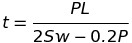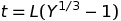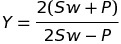# How To: Calculate The Thickness Of An Unstayed Full Hemispherical Head

When completing ASME code calculations involving cylindrical components it is possible during examinations you will be required to know how to calculate the thickness of an unstayed full hemispherical head. I recommend before working on questions involving full hemispherical heads, you read over the material and complete the practice questions regarding How To: Determine The Thickness Of A Blank Unstayed Dished Head.

Throughout this post I will provide and formula(s) and where to find them. Describe the variables used in the formula(s). Provide insight or sketches to help describe any of the code requirements I’ve found particularly important for understanding thickness of an unstayed full hemispherical head calculations. Then provide some practice questions to help reinforce the material, before wrapping everything up with a summary of the topic.

All page numbers referenced below are from the 2007 ASME Boiler & Pressure Vessel Code

## Formula

The formula for how to determine the thickness of an unstayed full hemispherical head with pressure on the concave side is under PG-29.11 page 14.

Minimum Thickness FormulaThe above equation should not be used when the required thickness of the head given by the above formula exceeds 35.6% of the inside radius, and instead these following two equations should be used:Where## Formula Variables

The formula variables above are explained on page 14 and are as follows:

L = Is the radius to which the head was formed, measures on the concave side of the head

P = Maximum allowable working pressure

S = Maximum allowable working stress, using values given in Table 1A of Section II, Part D

t = Minimum thickness of head

w = weld joint strength reduction factor per PG-26

## Important Full-Hemispherical Head Code Requirements

PG-29.12 page 14 – If a flanged-in manhole that meets the code requirements is placed in a full-hemispherical head, the thickness of the head shall be the same as for a head dished to a segment of a sphere ( see PG-29.1 and PG -29.5), with a dish radius equal to eight tenths the diameter of the shell and with the added thickness for the manhole as specified in PG-29.3.

## How To: Calculate The Thickness Of An Unstayed Full Hemispherical Head Questions

Note: All code questions are to be calculated in (mm) and (MPa) unless otherwise stated. Convert accordingly and properly before the calculation.

How To: Calculate The Thickness Of An Unstayed Full-Hemispherical Head Question #22

How To: Calculate  The Thickness Of An Unstayed Full-Hemispherical Head Question #23

## Summary